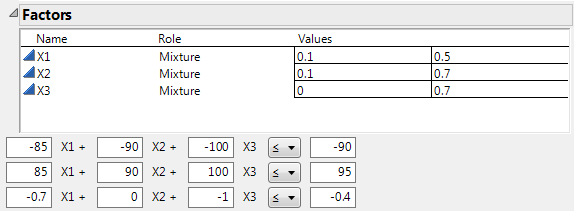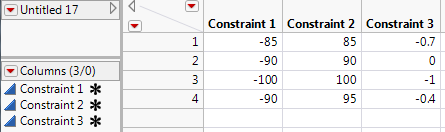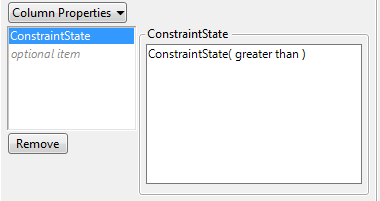Publication date: 07/30/2020

## ConstraintState Example

The sample data table Piepel.jmp, located in the Design Experiment folder, contains a mixture design with three continuous factors. The design is based on an experiment presented in Snee (1979) and Piepel (1988), where there are boundary constraints on each factor and three additional linear constraints. In the following example, you do the following:

1. Change one of the three additional constraints

2. Save the constraints to a table

3. Observe how the ConstraintState column property describes the direction of the inequality in the constraint

For further development of this example, see An Extreme Vertices Example with Linear Constraints in the Mixture Designs section.

1. Select Help > Sample Data Library and open Design Experiment/Piepel.jmp.

2. In the Table panel, click the green triangle next to the DOE Dialog script.

Notice the three linear constraints below the Factors outline. To make the constraints more interpretable, you want to reformulate the first constraint in terms of a “greater than or equal to” inequality.

Figure A.35 Linear Constraints beneath Factors Outline3. In the first constraint, do the following:

Type 85 next to X1.

Type 90 next to X2.

Type 100 next to X3.

Type 90 to the right of the inequality sign.

4. Click the Mixture Design red triangle and select Save Constraints.

A table containing information about the constraints appears.

Figure A.36 Constraint TableEach column contains the coefficients of the factors X1, X2, and X3 in rows 1 through 3. Row 4 contains the value that appeared to the right of the inequality sign.

5. Click the asterisk next to Constraint 1.

6. Click ConstraintState.

Figure A.37 ConstraintState Column Property PanelThe ConstraintState panel for X1 indicates that the direction of the inequality is “greater than” indicating greater than or equal to .

7. Click Cancel.